### APPENDIX 15: ORGANISATION OF OLYMPIC KATA COMPETITION

This Appendix 15 replaces Article 3: ORGANISATION OF KATA COMPETITION of the Kata Competition Rules for the purpose of Olympic Kata Competition. This Appendix 15 is based on the quota of 10 Competitors per category allowed by IOC for the 2020 Tokyo Olympic Games. Olympic Kata Competition is organised according the following procedure:

1. The 10 Competitors in each category are divided in 2 groups.
2. Each Competitor performs a first kata and receives the evaluation of the 7 judges.
3. Each Competitor performs a second kata and receives the evaluation of the 7 judges.
4. For each Competitor the average score of the two rounds are calculated.
5. Any ties are determined by the process described in this Appendix 15. The tie-break will not change the score registered.
6. In each of the two groups the two participants with the lowest score in their group is eliminated and the top three Competitors go on to a third round where the previous scores are disregarded.
7. In the third round the three Competitors in each of the two groups are given a new score for their third kata and deterring the ranking from 1 through 3 with the group.
8. In the fourth round, the medal bouts, the first place winners of the two groups are pitted against each other competing for 1st and 2nd place — and the Competitors placing second in their group are pitted against the Competitors placing third in the other group for competing for the 3rd places (bronze medals).
9. All other rules not specifically mentioned in this Appendix 15 are as per the Kata Competition Rules excluding Article 3.

#### EXPLANATION:

The following table illustrates the Organisation of Olympic Kata Competition: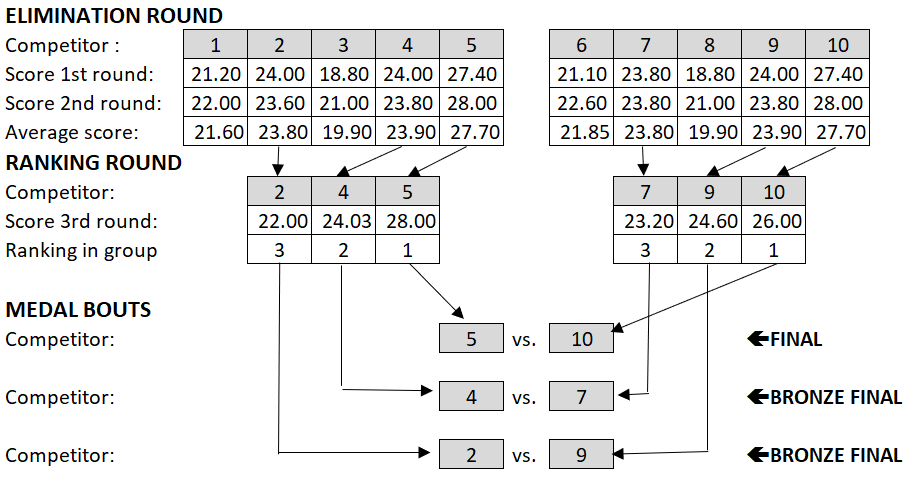#### Resolution of ties:

If 2 or more contestants have the same score, it will be considered several criteria to decide who have a better position in the ranking, following the criteria below.

#### Step 1:

Whoever has the highest score of the second kata in the elimination round wins. If the score in the second kata also are the same the subsequent steps are applied to the second kata preformed in the elimination round:

#### Step 2:

When the TOTAL SCORE of the second kata is equal, the following criteria will be considered:

the total score of the TECHNICAL CRITERIA before the multiplication for the factor (70%). The Highest win.

EXPLANATION

Example of equal score.

TOTAL SCORE
1. = 25.82
2. = 25.82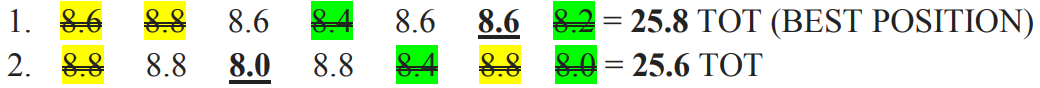In this case competitor N°. 1 has a better position in the competition ranking than competitor N° 2.

#### Step 3:

When the criteria considered in case 1 is the same we must consider the following:

the total score of the ATHLETIC CRITERIA before the multiplication for the factor (30%). The Highest win.

EXPLANATION

Example of equal score.

TOTAL SCORE
1. = 25.82
2. = 25.82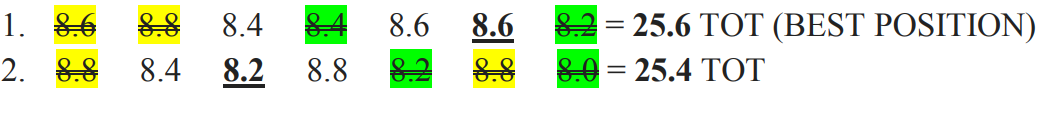In this case competitor N°. 1 has a better position in the competition ranking than competitor N° 2.

#### Step 4:

When the criteria considered in the previous cases are the same we must consider the following:

the score on the TECHNICAL CRITERIA, comparing the lowest score not excluded.
— The highest win.

EXPLANATION

Example of equal score.

TOTAL SCORE
1. = 25.82
2. = 25.82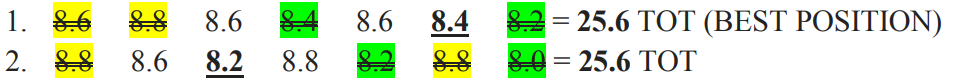In this case competitor N°. 1 has a better position in the competition ranking than competitor N° 2.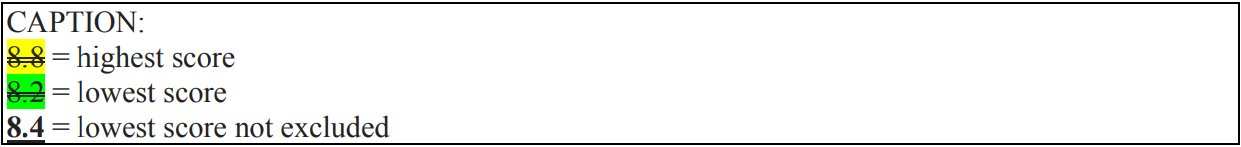#### Step 5:

When the criteria considered in the previous cases are the same we must consider the following:

the score on the TECHNICAL CRITERIA, comparing the highest score not excluded.
— The highest win.

EXPLANATION

Example of equal score.

TOTAL SCORE
1. = 25.82
2. = 25.82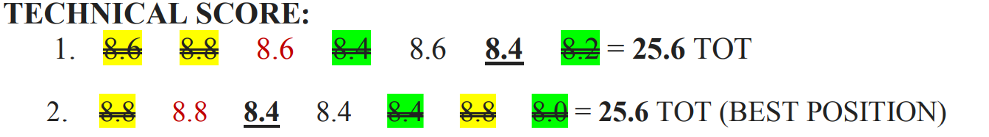In this case competitor N°. 2 will has a better position in the competition ranking than competitor N° 1.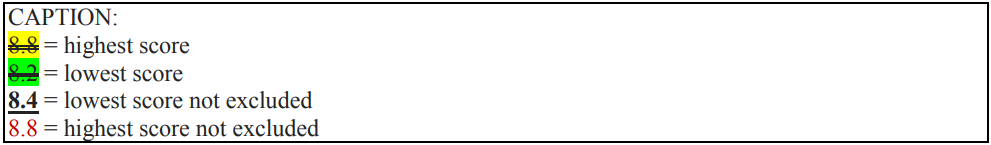#### Step 6:

When the criteria considered in the previous cases are the same we must consider the following:

the score on the ATHLETIC CRITERIA, comparing the lowest score not excluded.
— The highest win.

EXPLANATION

Example of equal score.

TOTAL SCORE
1. = 25.82
2. = 25.82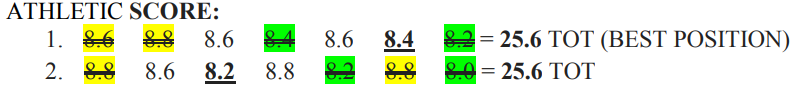In this case competitor N°. 1 has a better position in the competition ranking than competitor N° 2.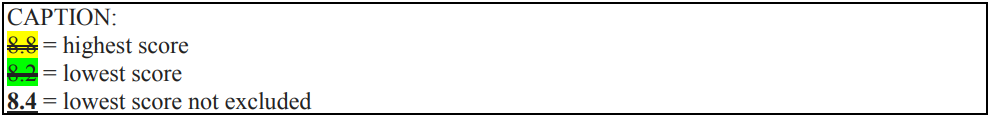#### Step 7:

When the criteria considered in the previous cases are the same we must consider the following:

the score on the ATHLETIC CRITERIA, comparing the highest score not excluded.
— The highest win.

EXPLANATION

Example of equal score.

TOTAL SCORE
1. = 25.82
2. = 25.82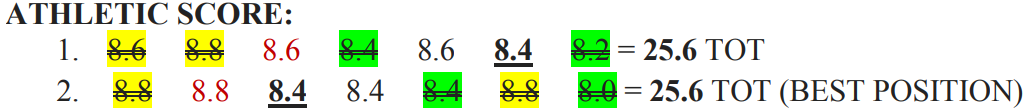In this case competitor N°. 2 will have a better position in the competition ranking than competitor N° 1.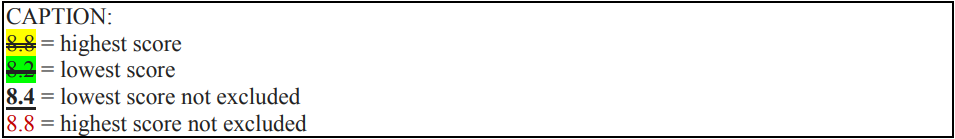#### Step 8:

When the criteria considered in the previous cases are the same we must consider the following:

the score on the TECHNICAL CRITERIA, comparing the highest score among the lowest scores excluded.

— The highest win.

EXPLANATION

Example of equal score.

TOTAL SCORE
1. = 25.82
2. = 25.82In this case competitor N°. 1 will have a better position in the competition ranking than competitor N° 2.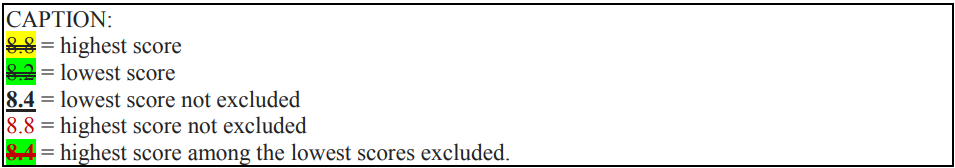#### Step 9:

When the criteria considered in the previous cases are the same we must consider the following:

the score on the TECHNICAL CRITERIA, comparing the lowest score among the highest scores excluded. The highest win.

EXPLANATION

Example of equal score.

TOTAL SCORE
1. = 25.82
2. = 25.82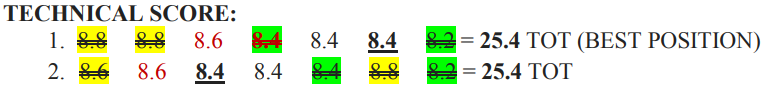In this case competitor N°. 1 will have a better position in the competition ranking than competitor N° 2.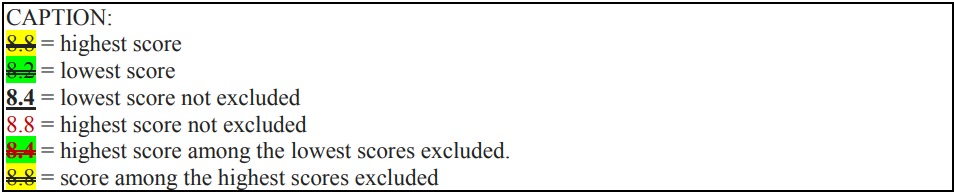#### Step 10:

When the criteria considered in the previous cases are the same we must consider the following:

the score on the TECHNICAL CRITERIA, comparing the lowest score among the lowest scores excluded. The highest win.

EXPLANATION

Example of equal score.

TOTAL SCORE
1. = 25.82
2. = 25.82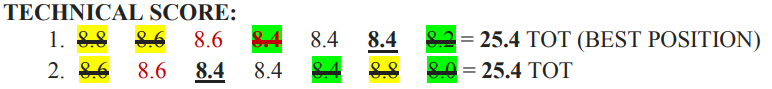In this case competitor N°. 1 will have a better position in the competition ranking than competitor N° 2.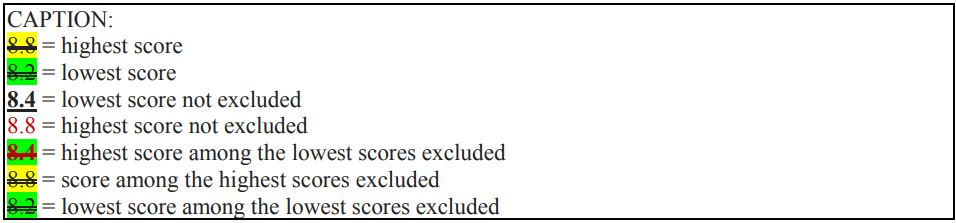#### Step 11:

When the criteria considered in the previous cases are the same we must consider the following:

the score on the ATHLETIC CRITERIA, comparing the highest score among the lowest scores excluded. The highest win.

EXPLANATION

Example of equal score.

TOTAL SCORE
1. = 25.82
2. = 25.82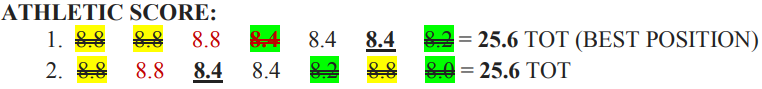In this case competitor N°. 1 will have a better position in the competition ranking than competitor N° 2.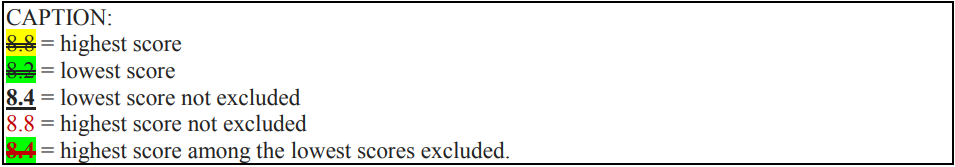#### Step 12:

When the criteria considered in the previous cases are the same we must consider the following:

the score on the ATHLETIC CRITERIA, comparing the lowest score among the highest scores excluded. The highest win.

EXPLANATION

Example of equal score.

TOTAL SCORE
1. = 25.82
2. = 25.82In this case competitor N°. 1 will have a better position in the competition ranking than competitor N° 2.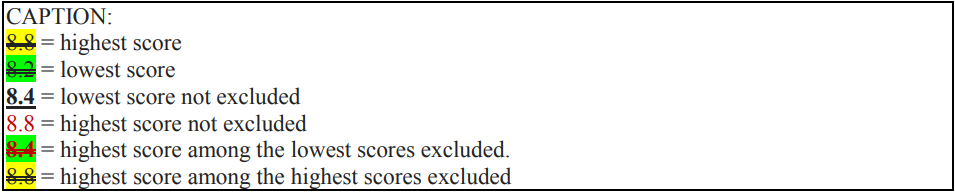#### Step 13:

When the criteria considered in the previous cases are the same we must consider the following: — the score on the ATHLETIC CRITERIA, comparing the lowest score among the lowest scores excluded. The highest win.

EXPLANATION

Example of equal score.

TOTAL SCORE
1. = 25.82
2. = 25.82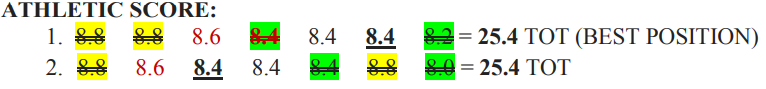In this case competitor N°. 1 will have a better position in the competition ranking than competitor N° 2.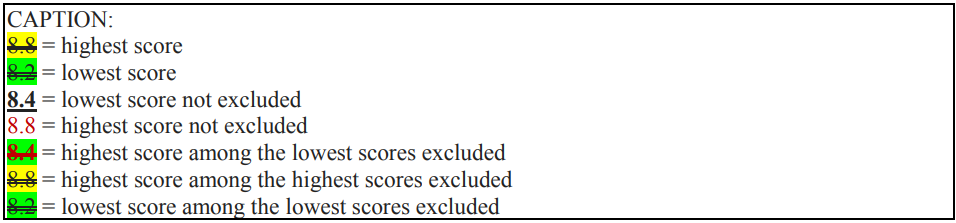#### Step 14:

When the criteria considered in the previous cases are the same we must consider the following:

the score on the TECHNICAL CRITERIA, comparing the highest score among the highest scores excluded. The highest win.

EXPLANATION

Example of equal score.

TOTAL SCORE
1. = 25.82
2. = 25.82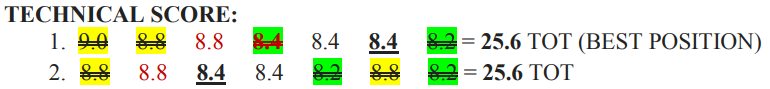In this case competitor N°. 1 will have a better position in the competition ranking than competitor N° 2.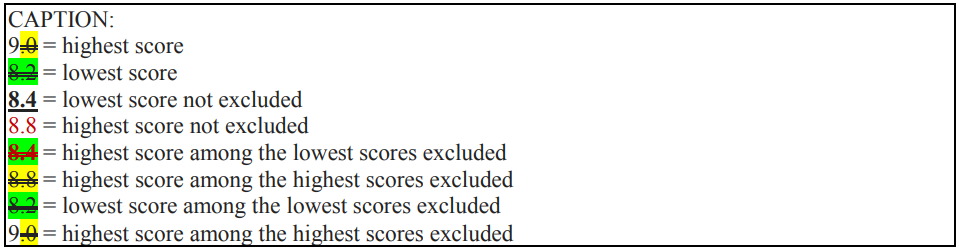#### Step 15:

When the criteria considered in the previous cases are the same we must consider the following:

the score on the ATHLETIC CRITERIA, comparing the highest score among the highest scores excluded. The highest win.

EXPLANATION

Example of equal score.

TOTAL SCORE
1. = 25.82
2. = 25.82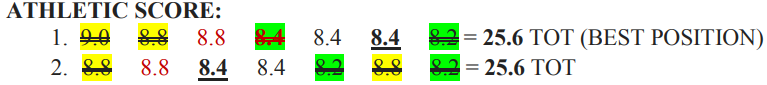In this case competitor N°. 1 will have a better position in the competition ranking than competitor N° 2.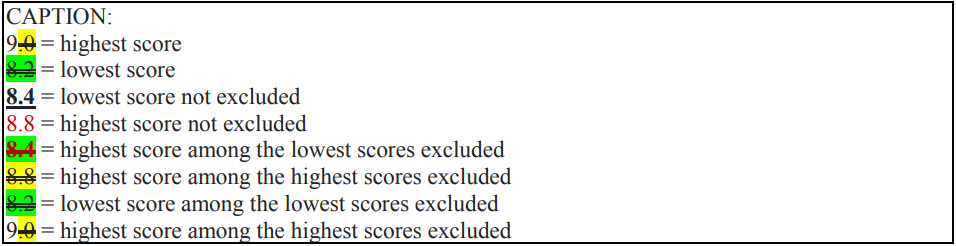#### Step 16:

When all the criteria considered in the previous cases are the same the tie will be resolved by coin toss.